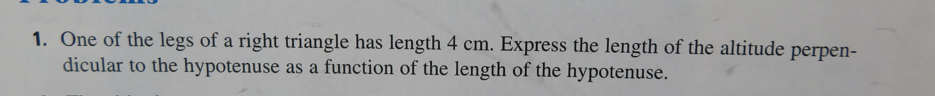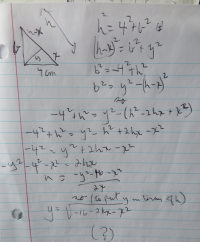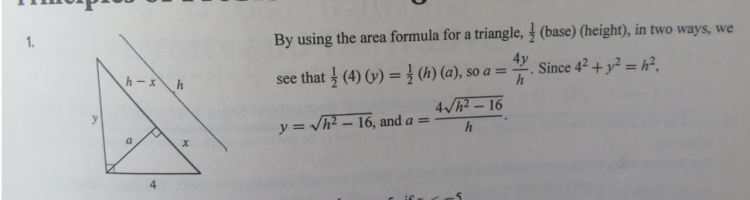a tough one

allegansveritatem

Full Member
Here is the problem:Here is the attempt I made:Actually I see that the final expression on the right should be squarerooted, but enough of that.

Now comes the solution given in the solutions manual. I have been puzzling over the this solution. Can't see how they go from the first step to the second. But here is the image:How do they get 1/2 4y=1/2 ha? Where does that come from?

jonah2.0

Full Member
Beer induced reaction follows.
...
How do they get 1/2 4y=1/2 ha? Where does that come from?
The solution explanation explicitly stated it.
"By using the area formula for a triangle, [imath]\frac{1}{2}[/imath](base)(height), in two ways, ..."
The left side used 4 as base & y as height.
The right side used h as base & a as height.

Jomo

Elite Member
They did not go from 4y/2 directly to ha/2! They calculated the area in two different ways and then equated those two expressions.

allegansveritatem

Full Member
Beer induced reaction follows.

The solution explanation explicitly stated it.
"By using the area formula for a triangle, [imath]\frac{1}{2}[/imath](base)(height), in two ways, ..."
The left side used 4 as base & y as height.
The right side used h as base & a as height.
A funny thing: After I posted this problem --- after an hour of fooling with it today---I sat down and got as still as I could and suddenly it came to me: The right side of the equation that had puzzled me in solutions manual was the formula for the area of THE SAME TRIANGLE that was being dealt with on the left side. Ha ha. I am ashamed to have called this a tough one! Somehow I couldn't get it through my head that the right side h and a were connected to the other two lines as a single entity. I saw two triangles but those two were not in the game...not as two anyway. Thanks for looking at this.

allegansveritatem

Full Member
They did not go from 4y/2 directly to ha/2! They calculated the area in two different ways and then equated those two expressions.
Yes, I see it now! Thanks

Jomo

Elite Member
A funny thing: After I posted this problem --- after an hour of fooling with it today---I sat down and got as still as I could and suddenly it came to me: The right side of the equation that had puzzled me in solutions manual was the formula for the area of THE SAME TRIANGLE that was being dealt with on the left side. Ha ha. I am ashamed to have called this a tough one! Somehow I couldn't get it through my head that the right side h and a were connected to the other two lines as a single entity. I saw two triangles but those two were not in the game...not as two anyway. Thanks for looking at this.
All math problems are tough until you figure out how to do it, then it becomes an easy problem.

jonah2.0

Full Member
Beer soaked query follows.
A funny thing: After I ...
Is that problem still from Swokowski's textbook?

allegansveritatem

Full Member
Beer soaked query follows.

Is that problem still from Swokowski's textbook?
No, this is from a calculus textbook, Zill and Wright, Early Transcendentals. I have just gotten through the first chapter which is all review. The problem in question is from the first section of the second chapter. It is a presentation of methods to solve problems based on the Polya system. The next section gets into calculus as one thinks of calculus. I think the first thing dealt with is limits.